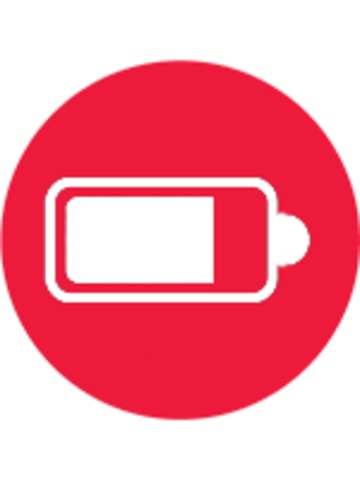EL233SBN

# 8 Digit Pocket Calculator

• Large 8 Digit display
• Battery powered
• Basic calculator functions plus memory, square root and percent
• Constant function
• Easy to use
POA## Pocket Calculator## LCD Display## Battery Operated## Auto Power Off• A compact pocket size calculator for general use, perfect for carrying in pants pockets or small bags for calculating all your daily mathematical equations including calculating tax amount
• LCD display showing up to 8 numbers at a time for convenience viewing.
• Storing the value of previous equation in memory.
• To calculate certain percentage of a number e.g. 100 + 10% gives you 110. Great function for calculating before or after tax amount of a certain item.
• "A portion of the equation is automatically memorised as a constant (function Plus, Minus, Division, Multiplication) , thus omitting redundant input. E.g. 2 x 5 = 10, pressing = will multiply by 5 again
• This function is great for instances where you'll need to calculate the same equation with a different variable e.g. stocktake or estimating sales.
• The square root of a number is a value that when multiplied by itself equals the original number. Great for jobs like architects, carpenters and engineers.
• The calculator powers off automatically when not in use to save battery.
Calculator Types
Pocket Calculators
Display
8 Digit LCD display
Dimensions (WxHxD)
60 x 103 x 8.3 millimetre
Memory
1
Percentage
Yes
Constant
Yes
Square Root
Yes
Auto Power Off
Yes